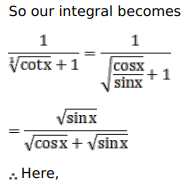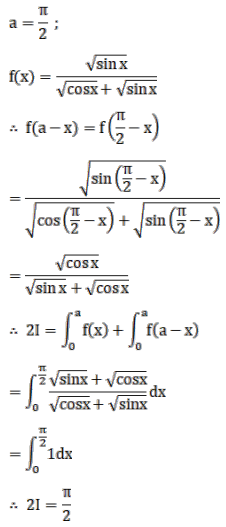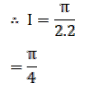# Mark against the correct answer in the following:

Question:

Mark $(\sqrt{)}$ against the correct answer in the following:

$\int_{0}^{\pi / 2} \frac{1}{(1+\sqrt{\cot x})} d x=?$

A. 0

B. $\frac{\pi}{4}$

C. $\frac{\pi}{2}$

D. $\pi$

Solution: